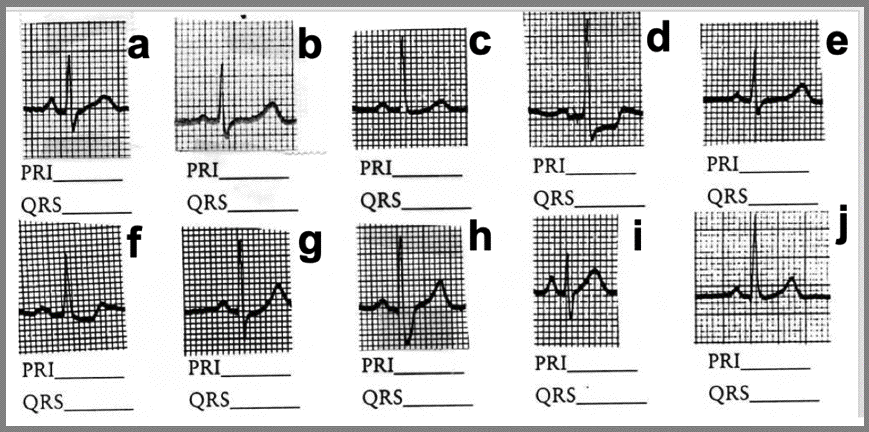### PRI and QRS Measurements: Please practice a LOT and you will get very good at these measurements...but you MUST PRACTICE! Also, for true accuracy go ahead and count 1/2 boxes if present. Quiz yourself at the bottom of this page!

The PRI measurement is from the point where the Pwave deflects from isoelectric to the beginning of the Qwave. If no Qwave is present, the end of the PRI is at the beginning of the Rwave. The QRS is from the beginning of the Qwave (or Rwave if no Qwave is present) to where the Swave returns to isoelectric (which is the J point).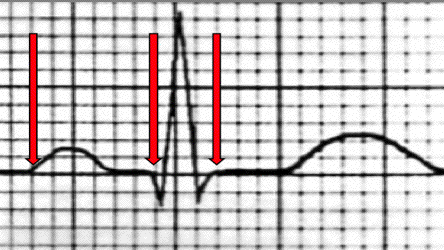The PRI above is 6 small boxes (.24 seconds) = prolonged
The QRS is 3 small boxes (.12 seconds) = normal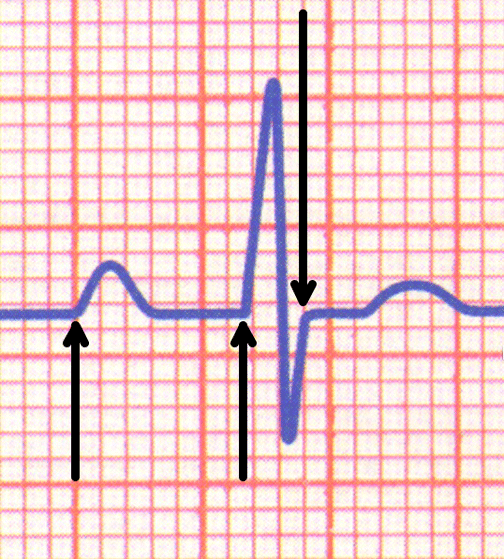The PRI above is 7 small boxes (.28 seconds) = prolonged
The QRS above is 2 small boxes (.08 seconds) = normalHow to Measure QRS when there is a Flat 'S' Wave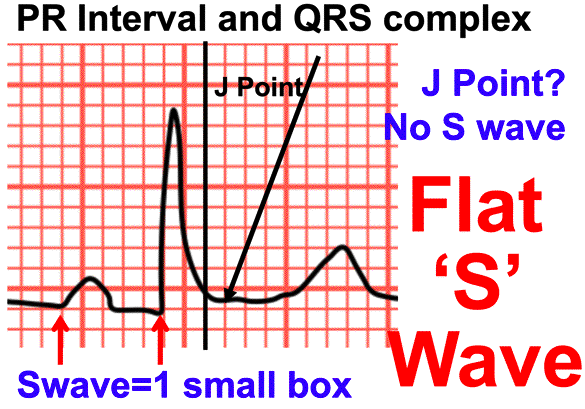In the PRI above there is no Q wave so going from the inflection of the Pwave to inflection of the Rwave you get a PRI of 5 small boxes (.2 seconds) = normal
The Flat 'S' wave poses a real challenge. Here are the steps:
1) Follow the Rwave until it reaches isoelectric or (as above) it starts to go toward the Twave
2) Mark the point where the Rwave begins to go towards the Twave
3) Move over 1 small box from your mark; that is the J point
4) You can now measure the QRS; for the above it is 3 small boxes (.12 seconds) = normal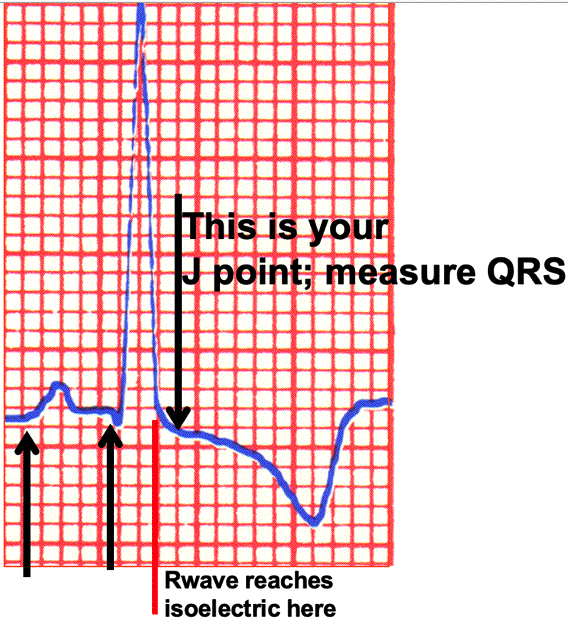The PRI above is 4.5 small boxes (.18 seconds) = normal
The Swave is not present due to the inverted Twave. Use the same technique as with the Flat 'S' Wave
1) Follow the Rwave until it reaches isoelectric: I have marked it with the red line
2) Move over 1 small box from where the Rwave reaches isoelectric: that is your J point
3) You can now measure the QRS; for the above it is 3.5 small boxes (.14 seconds) = abnormal
EKG PageQuiz to Check your Measurements: Mearure the PRI and QRS and classify. Check your work below.